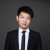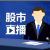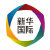0+0+0+0+0+0+0+0+0+0+0+0+0+0+0+0+0+0+0+0+0+0+0+0+0+0+0+0+0+0+#孟晚舟赢下一场法律战# 加拿大法院要求检方提交更多证据】据路透社11日报道，华为首席财务官孟晚舟的律师团队赢得了一场法律战，根据加拿大不列颠哥伦比亚省最高法院10日发布的一份裁决结果显示，法官要求加拿大司法部长交出更多与孟晚舟被捕有关的证据和文件。不列颠哥伦比亚省最高法院副首席法官...全文0+0+0+0+0+0+0+0+0+0+0+0+0+0+0+
Top